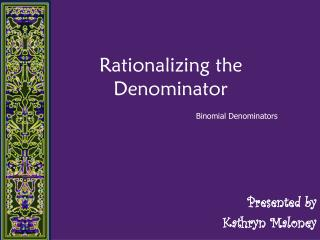DownloadDownload PresentationRationalizing the Denominator

# Rationalizing the Denominator

Télécharger la présentation## Rationalizing the Denominator

- - - - - - - - - - - - - - - - - - - - - - - - - - - E N D - - - - - - - - - - - - - - - - - - - - - - - - - - -
##### Presentation Transcript

1. Rationalizing the Denominator Binomial Denominators Presented by Kathryn Maloney

2. Rationalizing the Denominator (1) Multiply both the numerator and denominator by the conjugate of the denominator. (2) Simplify the result.

3. Cool Math Term Conjugate Surd A surd is a root that can’t be simplified further.

4. Product of Two Conjugates Product of the sum and difference of two terms. FOIL!

5. Perform the indicate division. (Rationalize the denominator) What is the conjugate of the denominator?

6. Perform the indicate division. (Rationalize the denominator) You Try! Press pause, find the solution, and play to check your answer!

7. Perform the indicate division. (Rationalize the denominator) What is the conjugate of the denominator? You Try!

8. Perform the indicate division. (Rationalize the denominator) What is the conjugate of the denominator? 4 FOIL

9. Perform the indicate division. (Rationalize the denominator) You Try! Press pause, find the solution, and play to check your answer!

10. Perform the indicate division. (Rationalize the denominator) What is the conjugate of the denominator? 1 You Try! FOIL

11. Perform the indicate division. (Rationalize the denominator) What is the conjugate of the denominator? FOIL

12. Perform the indicate division. (Rationalize the denominator) You Try! Press pause, find the solution, and play to check your answer!

13. Perform the indicate division. (Rationalize the denominator) What is the conjugate of the denominator? You Try! FOIL

14. Thank you! Don't worry about moving slowly, worry about standing still. -- Chinese proverb